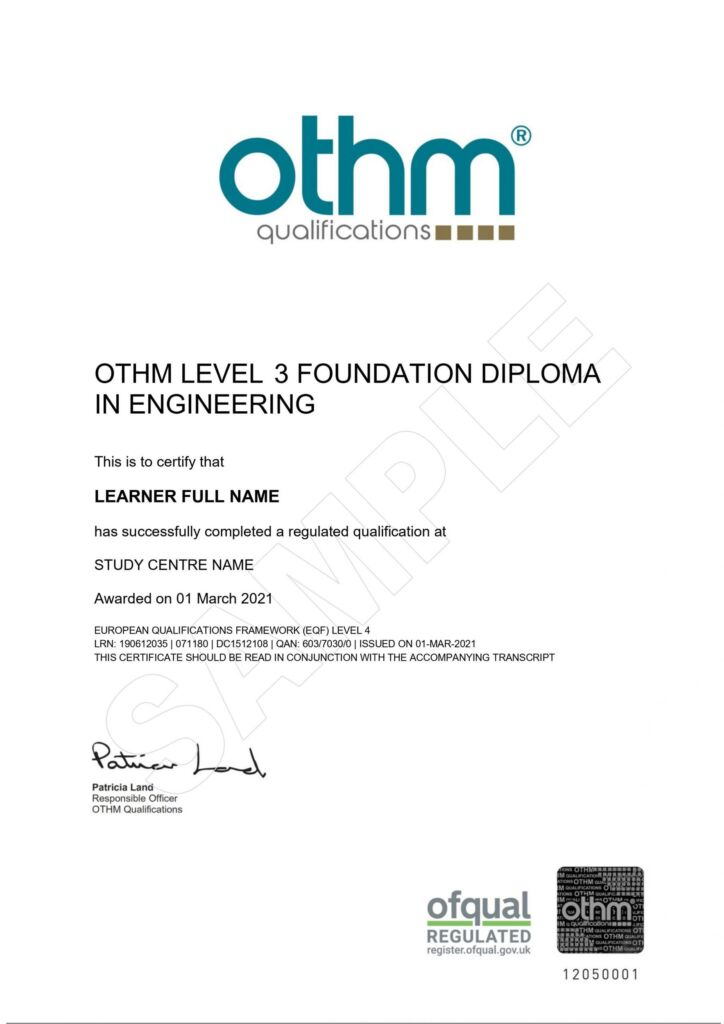## Engineering (60 Credits)

Course Includes

• University Undergraduate (Level 3) Full Course Materials and Assignments

Also includes

• Unlimited tutor support
• Recognised Ofqual Qualification

Do you want to pursue a career in Engineering? Engineering isn’t all about construction and building sites; it can lead to a career in the medical field or economics – it is a widely diverse profession to choose. Our Level 3 Foundation Diploma in Engineering allows learners to gain understanding and knowledge across a range of Engineering practices.

This qualification enables learners to develop essential knowledge in areas such as engineering principles, design technology, electricity, mechanics, statistics and mathematics.

Successful completion of this qualification will prepare learners for employment in the engineering sector or further learning.

## Examples of University Progression

• Southampton Solent University
• University of Derby
• University of Lincoln
• University of Central Lancashire (UCLan)

## Eligibility Criteria

This course is most suited to individuals who are at least 16 years old, have a high school education and wish to progress onto a degree at university.

## Awarding BodyEngineering Principles

• Understand applications of SI units and measurement.
• Know how to perform engineering calculations.
• Know how to interpret engineering information.

Design Technology

• Demonstrate knowledge and understanding of engineering products and design.
• Understand how to apply a systems approach to electrical design.
• Be able to produce 2D CAD drawings.

Electricity

• Understand the functions of basic electrical components within circuits.
• Be able to apply AC and DC circuit theory to circuit design.
• Understand power supplies and power system protection.

Mechanics

• Understand the motion of objects in mathematical terms.
• Understand energy changes in a system.
• Understand energy transfer, forces and elasticity.

Introducing Statistics

• Understand the underpinning concepts relating to the analysis of statistics.
• Understand a range of data types and their representation.
• Be able to calculate and interpret statistical diagrams and measures.

Mathematics

• Understand the application of algebra relevant to engineering problems.
• Be able to use geometry and graphs in the context of engineering problems.
• Understand exponentials, logarithms and trigonometry related to engineering problems.
• Understand calculus relevant to engineering problems.

## Written Assignments

The Level 3 Foundation Diploma in Engineering has 6 modules and 6 written assignments. On completion of the modules, students will be given access to the assignments. The assignments are approximately 5,000-8,000 words each. Students are provided support on the modules and assignments via the ‘Tutor’ section of the learning platform.

The assignment unit titles that students need to complete for the Level 3 course are:

1. Engineering Principles
2. Design Technology
3. Electricity
4. Mechanics
5. Introducing Statistics
6. Mathematics